Open in App
Not now

# How to apply CSS page-break to print a table with lots of rows?

• Last Updated : 19 Aug, 2020

When printing a table with lots of rows the problem can arise in keeping the data together when the page ends. Since the data presented continuously makes more sense. Here we will track down ways by which one can print contents of a table with lots of rows when the situation of page-break is encountered.

The most logical property that can be used for this purpose is page-break in CSS.

page-break in CSS
It is CSS property that help to define how a elements on a page will look when printed. This makes the print of the document more book-like.
page-break isn’t a directly usable property but it contains three properties that can be used as per requirement:

1. page-break-before: adds a page break before an element
2. page-break-after: adds a page break after an element
3. page-break-inside: sets whether the page break should be avoided or not inside an element.

Syntax:

`name_of_the_element { name_of_the_property: value;}`

Example:

`table {page-break-before: always;}`

Here, name_of_the_element refers to the element page break is required on. (eg., table)
name_of_the_property refers to the property that is required to apply to the element. (eg., page-break-before)
value dictates how the given property is supposed to behave when the document is printed. (eg., always)

It can be applied wherever required, inside the table, before or after the table or before or after a row, and even within a row. But they should be applied such that the formatted output makes sense in a hard copy.

The following programs will help you understand better. Their output can be seen only when printed thus pdf has been attached.

Program 1: program that takes new page when a table starts

 ```<``html``>``<``head``>``    ``<``title``>``    ``<``style` `type``=``"text/css"``>``        ``table {page-break-before: always;}``    ``````<``body``>``    ``hello from the Geeksforgeeks``    ``<``table``>``        ``<``tr``>``            ``<``th``>s.no.``            ``<``th``>name``        ````        ``<``tr``>``            ``<``td``> 1 ``            ``<``td``> apple ``        ````        ``<``tr``>``            ``<``td``> 2 ``            ``<``td``> mango ``        ````    ```````

Output:
page1: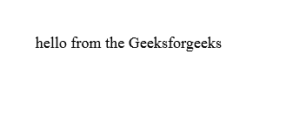page2: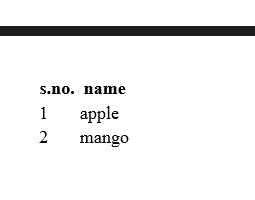Program 2: program that takes a new page when a table starts and when a new page is required while printing rows but not in between rows.

 ```<``html``>``<``head``>``    ``<``title``>``    ``<``style` `type``=``"text/css"``>``        ``table {page-break-before: always; ``               ``font-size: 100px;}``        ``tr{page-break-inside: avoid; ``           ``page-break-after: auto;}``    ``````<``body``>``    ``hello from the Geeksforgeeks``    ``<``table``>``        ``<``tr``>``            ``<``th``>s.no.``            ``<``th``>name``        ````        ``<``tr``>``            ``<``td``> 1 ``            ``<``td``> apple ``        ````        ``<``tr``>``            ``<``td``> 2 ``            ``<``td``> mango ``        ````        ``<``tr``>``            ``<``td``> 3 ``            ``<``td``> kiwi ``        ````        ``<``tr``>``            ``<``td``> 4 ``            ``<``td``> banana ``        ``  ``        ``<``tr``>``            ``<``td``> 5 ``            ``<``td``> strawberry ``        `` ``        ``<``tr``>``            ``<``td``> 6 ``            ``<``td``> guava ``        `` ``        ``<``tr``>``            ``<``td``> 7 ``            ``<``td``> watermelon ``        ``    ``    ```````

Output:
page1: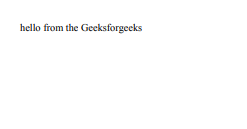page2: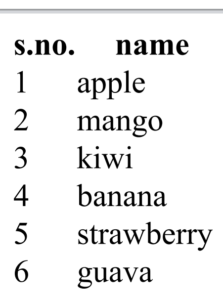page3: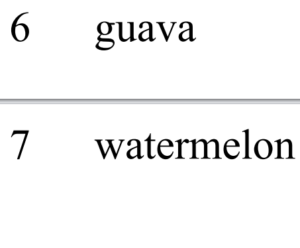All these outputs will be generated when printed, the outputs attached above are screenshots of print preview.

My Personal Notes arrow_drop_up